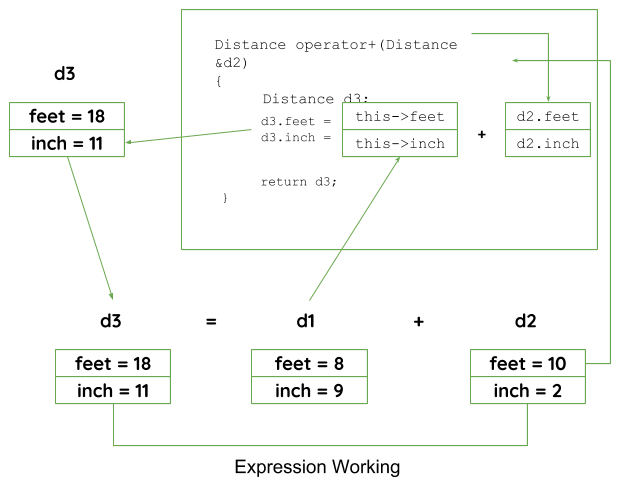Open In App
Related Articles
• Write an Interview Experience
• C++ Programming Language

C++ provides a special function to change the current functionality of some operators within its class which is often called as operator overloading. Operator Overloading is the method by which we can change the function of some specific operators to do some different tasks.

Syntax:

```Return_Type classname :: operator op(Argument list)
{
Function Body
}// This can be done by declaring the function```

Here,

• Return_Type is the value type to be returned to another object.
• operator op is the function where the operator is a keyword.
• op is the operator to be overloaded.

## Criteria/Rules to Define the Operator Function

1. In the case of a non-static member function, the binary operator should have only one argument and the unary should not have an argument.
2. In the case of a friend function, the binary operator should have only two arguments and the unary should have only one argument.
3. All the class member objects should be public if operator overloading is implemented.
4. Operators that cannot be overloaded are  .* :: ?:
5. Operators that cannot be overloaded when declaring that function as friend function are = () [] ->.
6. The operator function must be either a non-static (member function) or a friend function.

Let us consider overloading (-) unary operator. In the unary operator function, no arguments should be passed. It works only with one class object. It is the overloading of an operator operating on a single operand.

Example: Assume that class Distance takes two member objects i.e. feet and inches, and creates a function by which the Distance object should decrement the value of feet and inches by 1 (having a single operand of Distance Type).

## C++

 `// C++ program to show unary ``// operator overloading``#include ``using` `namespace` `std;`` ` `class` `Distance {``public``:``    ``int` `feet, inch;`` ` `    ``// Constructor to initialize ``    ``// the object's value``    ``Distance(``int` `f, ``int` `i)``    ``{``        ``this``->feet = f;``        ``this``->inch = i;``    ``}`` ` `    ``// Overloading(-) operator to ``    ``// perform decrement operation ``    ``// of Distance object``    ``void` `operator-()``    ``{``        ``feet--;``        ``inch--;``        ``cout << ``"\nFeet & Inches(Decrement): "` `<< ``                  ``feet << ``"'"` `<< inch;``    ``}``};`` ` `// Driver Code``int` `main()``{``    ``Distance d1(8, 9);`` ` `    ``// Use (-) unary operator by ``    ``// single operand``    ``-d1;``    ``return` `0;``}`

Output

`Feet & Inches(Decrement): 7'8`

Explanation: In the above program, it shows that no argument is passed and no return_type value is returned, because the unary operator works on a single operand. (-) operator changes the functionality to its member function.

Note: d2 = -d1 will not work, because operator-() does not return any value.

In the binary operator overloading function, there should be one argument to be passed. It is the overloading of an operator operating on two operands. Below is the C++ program to show the overloading of the binary operator (+) using a class Distance with two distant objects.

## C++

 `// C++ program to show binary ``// operator overloading``#include ``using` `namespace` `std;`` ` `class` `Distance {``public``:``    ``int` `feet, inch;``   ` `    ``Distance()``    ``{``        ``this``->feet = 0;``        ``this``->inch = 0;``    ``}`` ` `    ``Distance(``int` `f, ``int` `i)``    ``{``        ``this``->feet = f;``        ``this``->inch = i;``    ``}`` ` `    ``// Overloading (+) operator to ``    ``// perform addition of two distance ``    ``// object``    ``// Call by reference``    ``Distance operator+(Distance& d2) ``    ``{``        ``// Create an object to return``        ``Distance d3;`` ` `         ` `        ``d3.feet = ``this``->feet + d2.feet;``        ``d3.inch = ``this``->inch + d2.inch;`` ` `        ``// Return the resulting object``        ``return` `d3;``    ``}``};`` ` `// Driver Code``int` `main()``{``    ``Distance d1(8, 9);``    ``Distance d2(10, 2);``    ``Distance d3;`` ` `    ``// Use overloaded operator``    ``d3 = d1 + d2;`` ` `    ``cout << ``"\nTotal Feet & Inches: "` `<< ``             ``d3.feet << ``"'"` `<< d3.inch;``    ``return` `0;``}`

Output

`Total Feet & Inches: 18'11`

Explanation:

1. Line 27, Distance operator+(Distance &d2): Here return type of function is distance and it uses call by references to pass an argument.
2. Line 49, d3 = d1 + d2: Here, d1 calls the operator function of its class object and takes d2 as a parameter, by which the operator function returns the object and the result will reflect in the d3 object.

### Pictorial View of working of Binary OperatorIn this approach, the operator overloading function must be preceded by the friend keyword, and declare the function in the class scope. Keeping in mind, the friend operator function takes two parameters in a binary operator and varies one parameter in a unary operator. All the working and implementation would same as the binary operator function except this function will be implemented outside the class scope.

Example: Below is the C++ program to show binary operator overloading using a friend function.

## C++

 `// C++ program to show binary ``// operator overloading using ``// a Friend Function``#include ``using` `namespace` `std;`` ` `class` `Distance {``public``:``   ` `    ``int` `feet, inch;`` ` `    ``Distance()``    ``{``        ``this``->feet = 0;``        ``this``->inch = 0;``    ``}`` ` `    ``Distance(``int` `f, ``int` `i)``    ``{``        ``this``->feet = f;``        ``this``->inch = i;``    ``}`` ` `    ``// Declaring friend function ``    ``// using friend keyword``    ``friend` `Distance operator + (Distance&, ``                                ``Distance&);``};`` ` `// Implementing friend function ``// with two parameters``// Call by reference``Distance operator+(Distance& d1,``                   ``Distance& d2) ``{``    ``// Create an object to return``    ``Distance d3;`` ` `    ``d3.feet = d1.feet + d2.feet;``    ``d3.inch = d1.inch + d2.inch;`` ` `    ``// Return the resulting object``    ``return` `d3;``}`` ` `// Driver Code``int` `main()``{``    ``Distance d1(8, 9);``    ``Distance d2(10, 2);``    ``Distance d3;`` ` `    ``// Use overloaded operator``    ``d3 = d1 + d2;`` ` `    ``cout << ``"\nTotal Feet & Inches: "` `<< ``             ``d3.feet << ``"'"` `<< d3.inch;``    ``return` `0;``}`

Output

`Total Feet & Inches: 18'11`

Explanation: The operator function is implemented outside of the class scope by declaring that function as the friend function.

In these ways, an operator can be overloaded to perform certain tasks by changing the functionality of operators.